• 改进的有效边表算法
千次阅读
2017-04-22 17:12:54

这里不仔细讲原理，只是把我写的算法发出来，跟大家分享下，如果有错误的话，还请大家告诉我，如果写的不好，也请指出来，一起讨论进步。

边表构造的算法:

(1) 首先构造一个纵向链表，链表的长度为多边形所占有的最大扫描线数，链表的每个结点，称为一个桶，则对应多边形覆盖的每一条扫描线。

(2) 将每条边的信息链入与该边最小y坐标相对的桶处。也就是说，若某条边的较低点为ymin，则该边就放在相应的扫描线中。

(3) 每条边的数据形成一个结点，内容包括:该扫描线与该的初始交点x(即较低端点的x值),1/k，以及该边的最大y值ymax如下：

x|ymin　　ymax　　1/k 　　next

(4) 同一桶中若干条边按x|ymin由小到在大排序，若x|ymin相等，则按照1/k由小到大排序。

改进的有效边表填充算法步骤如下:

(1) 初始化： 构造边表， AET表置空.

(2) 将第一个不空的ET表中的边与AET表合并。

(3) 删除AET表中y = ymax的边后再填充，按“下闭上升”的原则进行填充，此时就无需缩短任何边。然后进行填充，填充时设置一个布尔量b(初值为假)，令指针从有效边表中第一个结点到最后一个结点进行遍历一次。每访问一个结点，把b取反一次，若b为真，则把从当前结点的x值开始下一结点的x值结束的区间用多边形色填充。(填充时需要对x坐标进行四舍五入处理)。

(4) yi+1 = yi + 1,根据xi+1 = x+ 1 / k计算并修改AET表，同时合并ET表中y = yi+1桶的边，按次序插入到AET表中，形成新的AET表。

(5) AET表不空则转(3)，否则结束。

/*
*	Date: 11/23/2010
*/
#include <GL/freeglut.h>
#include <iostream>
#include <vector>
#include <fstream>
std::ifstream cin ("polypoints.txt");
using std::vector;
using std::endl;
typedef struct _EdgeItem
{
float	x;
int		yMax;
float	reverseK;					// 1/k
_EdgeItem * next;
}EdgeItem;
vector<EdgeItem *> g_pItemVector;
typedef struct _Point
{
int x;
int y;
}Point;

typedef struct _EdgePtr
{
int		nScanLine;					// Current scan line
EdgeItem * pItem;					// Pointer to edge item
}EdgePtr;

typedef struct _PolyPoints
{
Point * pPoint;						// Pointer to points
int		n;							// Number of points
int		yMax;						// Max y of all points
int		yMin;						// Min y of all points
}PolyPoints;

EdgePtr * g_pEdgeList;					// Edge list
EdgePtr * g_pActiveEdgeList;				// Active edge list
PolyPoints g_polyPoints;				// Storage for points of polygons

void inputPoints (void)
{
int n;
cin>>n;
if (n < 3)
{
std::cout<<"number of points can not be less than 3"<<endl;
exit (0);
}
g_polyPoints.n = n;
g_polyPoints.pPoint = new Point[n];
g_polyPoints.yMax = INT_MIN;
g_polyPoints.yMin = INT_MAX;
int x, y;
for (int i = 0; i < n; ++i)
{
cin>>x>>y;
g_polyPoints.pPoint[i].x = x;
g_polyPoints.pPoint[i].y = y;
if (g_polyPoints.yMax < y)
{
g_polyPoints.yMax = y;
}
if (g_polyPoints.yMin > y)
{
g_polyPoints.yMin = y;
}
}

}
// Calculate the reverse of k
float calculateReverseK (const Point & p1, const Point & p2)
{
return (float)(p2.x - p1.x) / (float)(p2.y - p1.y);
}

// Sort one scan line's list, first sort by x, if x is equal then sort by 1/k
void sortOneScanLineEdgeList (EdgePtr & edgePtr)
{
// Sort by x (select sort)
EdgeItem * pEdgeItem = edgePtr.pItem;
EdgeItem * pNext;
EdgeItem * pTmp;
while (pEdgeItem)
{
pNext = pEdgeItem->next;
pTmp = pEdgeItem;
while (pNext)
{
if (pNext->x < pTmp->x)
{
pTmp = pNext;
}
pNext = pNext->next;
}
if (pTmp != pEdgeItem)
{
// Swap x
float fTmp = pTmp->x;
pTmp->x = pEdgeItem->x;
pEdgeItem->x = fTmp;
// Swap yMax

int iTmp = pTmp->yMax;
pTmp->yMax = pEdgeItem->yMax;
pEdgeItem->yMax = iTmp;
// Swap 1/k
float kTmp = pTmp->reverseK;
pTmp->reverseK = pEdgeItem->reverseK;
pEdgeItem->reverseK = kTmp;
}
pEdgeItem = pEdgeItem->next;
}
// When the x is the same, then sort by 1/k
pEdgeItem = edgePtr.pItem;
EdgeItem * pStart = NULL;
EdgeItem * pEnd = NULL;
while (pEdgeItem)
{
// Find the start pointer and end pointer with the same x, then sort them
pEnd = pStart = pEdgeItem;
pNext = pStart->next;
while (pNext && (pNext->x == pStart->x))
{
pEnd = pNext;
pNext = pNext->next;
}
// Sort the edge list from pStart to pEnd by 1/k (select sort)
while (pStart != pEnd)
{
pTmp = pStart;
pNext = pTmp->next;
while (pNext != pEnd)
{
if (pNext->reverseK < pTmp->reverseK)
{
pTmp = pNext;
}
pNext = pNext->next;
}
// Swap values
if (pTmp != pStart)
{
// Swap x
float fTmp = pTmp->x;
pTmp->x = pStart->x;
pStart->x = fTmp;
// Swap yMax
int iTmp = pTmp->yMax;
pTmp->yMax = pStart->yMax;
pStart->yMax = iTmp;
// Swap 1/k
float kTmp = pTmp->reverseK;
pTmp->reverseK = pStart->reverseK;
pStart->reverseK = kTmp;

}
pStart = pStart->next;
} // while (pStart != pEnd)
pEdgeItem = pEnd->next;
}
}
// Construct the edge list
void constructEdgeList (void)
{
// Construct the edge list
int nScanLines = g_polyPoints.yMax - g_polyPoints.yMin + 1;
g_pEdgeList = new EdgePtr[nScanLines];
memset (g_pEdgeList, 0, sizeof (EdgePtr) * nScanLines);
Point * pPoint = g_polyPoints.pPoint;
int nScanLine = g_polyPoints.yMin;

EdgeItem * pEdgeItem = NULL;
for (int i = 0; i < nScanLines; ++i, ++ nScanLine)
{
g_pEdgeList[i].nScanLine = nScanLine;
for (int j = 0; j < g_polyPoints.n; ++j)
{
if (pPoint[j].y == nScanLine)
{
int j1 = (j+g_polyPoints.n-1) % g_polyPoints.n;
int j2 = (j+1) % g_polyPoints.n;
// if point j1's y > nScanLine then add this edge to the current scanline's list
if (pPoint[j1].y > nScanLine)
{
pEdgeItem = new EdgeItem;
pEdgeItem->reverseK = calculateReverseK (pPoint[j], pPoint[j1]);
pEdgeItem->x = (float)pPoint[j].x;
pEdgeItem->yMax = pPoint[j1].y;
// Add pEdgeItem to the scanline's list
pEdgeItem->next = g_pEdgeList[i].pItem;
g_pEdgeList[i].pItem = pEdgeItem;

}
// if point j2's y > nScanLine then add this edge to the curretn scanline's list
if (pPoint[j2].y > nScanLine)
{
pEdgeItem = new EdgeItem;
pEdgeItem->reverseK = calculateReverseK (pPoint[j], pPoint[j2]);
pEdgeItem->x = (float)pPoint[j].x;
pEdgeItem->yMax = pPoint[j2].y;
// Add pEdgeItem to the scanline's list
pEdgeItem->next = g_pEdgeList[i].pItem;
g_pEdgeList[i].pItem = pEdgeItem;
}
} // if (pPoints[j].y == nScanLine)
} // for (int j = 0; j < g_polyPoints.n; ++j)
sortOneScanLineEdgeList (g_pEdgeList[i]);
}
// Init the active edge list
g_pActiveEdgeList = new EdgePtr[nScanLines];
}
// free the memory
void destroy (void)
{
if (g_pActiveEdgeList)
{
delete g_pActiveEdgeList;
}
int nScanLines = g_polyPoints.yMax - g_polyPoints.yMin + 1;
EdgeItem * pItem, * pNext;
if (g_pEdgeList)
{
for (int i = 0; i < nScanLines; ++i)
{
if (g_pEdgeList[i].pItem)
{
pItem = g_pEdgeList[i].pItem;
pNext = pItem;
while (pItem)
{
pNext = pItem->next;
delete pItem;
pItem = pNext;
}
}
}
}
}
void init (void)
{
glClearColor (0.0f, 0.0f, 0.0f, 1.0f);
}

void activEdgeListFillPolygon (void)
{
int nScanLines = g_polyPoints.yMax - g_polyPoints.yMin + 1;
memset (g_pActiveEdgeList, 0, sizeof (EdgePtr) * nScanLines);
int nScanLine = g_polyPoints.yMin;
glBegin (GL_POINTS);
int i = 0;
for (;i < nScanLines;  ++ nScanLine, ++ i)
{
if (g_pEdgeList[i].pItem)
{
g_pActiveEdgeList[i].pItem = g_pEdgeList[i].pItem;
break;
}
}
for (int j = i; j < nScanLines; ++j, ++ nScanLine)
{
if (g_pActiveEdgeList[j].pItem)
{
// Delete the edge where yMax = nScanLine
EdgeItem * pPre = NULL;
EdgeItem * pNext = g_pActiveEdgeList[j].pItem;
bool bEven = true;
while (pNext)
{
if (pNext->yMax == nScanLine)
{
if (!pPre)
{
g_pActiveEdgeList[j].pItem = pNext->next;
pNext = pNext->next;
}
else
{
pPre->next = pNext->next;
pNext = pNext->next;
}
}
else
{
bEven = !bEven;
pPre = pNext;
pNext = pNext->next;
}
} // while (pNext)

// Fill the scan line when bFill is true
bool bFill = false;
pNext = g_pActiveEdgeList[j].pItem;
while (pNext && bEven)
{
bFill = !bFill;
if (bFill)
{
int x1 = (int)(pNext->x + 0.5);
int x2 = (int)(pNext->next->x + 0.5);
//int x1 = pNext->x;
//int x2 = pNext->next->x;
for (int i = x1; i <= x2; ++i)
{
glVertex2i (i, nScanLine);
}
}
pNext = pNext->next;
}	// while (pNext)
pNext = g_pActiveEdgeList[j].pItem;
int kk = j + 1;
if (kk < nScanLines)
{
while (pNext)
{
EdgeItem * pItem = new EdgeItem;
pItem->x = pNext->x + pNext->reverseK;
pItem->reverseK = pNext->reverseK;
pItem->yMax = pNext->yMax;
pItem->next = g_pActiveEdgeList[kk].pItem;
g_pActiveEdgeList[kk].pItem = pItem;
pNext = pNext->next;
g_pItemVector.push_back (pItem);
} // while (pNext)
// Add edge list to active edge list
pNext = g_pEdgeList[kk].pItem;
EdgeItem * pTemp = NULL;
while (pNext)
{
pTemp = new EdgeItem;
pTemp->reverseK = pNext->reverseK;
pTemp->x = pNext->x;
pTemp->yMax =pNext->yMax;
g_pItemVector.push_back (pTemp);
pTemp->next = g_pActiveEdgeList[kk].pItem;
g_pActiveEdgeList[kk].pItem = pTemp;
pNext = pNext->next;
}
sortOneScanLineEdgeList (g_pActiveEdgeList[kk]);
}

} // 			if (g_pActiveEdgeList[j].pItem)
}
glEnd ();
　　　　// 这里为了简单所以把分配的内存放在vector容器中，方便删除。:-)
vector<EdgeItem *>::iterator itr = g_pItemVector.begin (),
endItr = g_pItemVector.end ();
while (itr != endItr)
{
delete (*itr);
++ itr;
}
g_pItemVector.clear ();
}

void display (void)
{
glClear (GL_COLOR_BUFFER_BIT);
glColor3f (1.0f, 0.0f, 0.0f);
// Fill a polygon
activEdgeListFillPolygon ();
glutSwapBuffers ();
}

void reshape (int w, int h)
{
glViewport (0, 0, (GLsizei) w, (GLsizei) h);
glMatrixMode (GL_PROJECTION);
if (w <= h)
{
gluOrtho2D (-600.0, 600.0, -600.0 * (GLfloat) h / (GLfloat) w, 600.0 * (GLfloat) h / (GLfloat) w);
}
else
{
gluOrtho2D (-600.0 * (GLfloat) w / (GLfloat) h,600.0 * (GLfloat) w / (GLfloat) h, -600.0, 600.0);
}
glMatrixMode (GL_MODELVIEW);
}
void keyboard (unsigned char key, int x, int y)
{
switch (key)
{
case 27: // 'VK_ESCAPE'
		destroy ();
　　　　　　exit (0);
break;
default:
break;
}
}
int main (int argc, char ** argv)
{
glutInit (&argc, argv);
glutInitDisplayMode (GLUT_DOUBLE | GLUT_RGB);
glutInitWindowSize (600, 600);
glutCreateWindow ("Optimized active edge list");
init ();
inputPoints ();
constructEdgeList ();

glutReshapeFunc (reshape);
glutDisplayFunc (display);
glutKeyboardFunc (keyboard);
glutMainLoop ();
destroy ();   // 这里destroy并没有调用。
return 0;
}


polypoints.txt
中的示例内容如下:
7
30 120
10 70
30 10
60 50
80 10
120 90
70 80
更多相关内容
• 实验二 有效边表填充算法 实验题目 有效边表填充算法 姓名 指导老师 学号 班级 完成日期 1.实验目的 设计有效边表结点和边结点数据结构 设计有效边表填充算法 编程实现有效边表填充算法 2.实验描述 下图 1 所示...
• 改进有效边表算法，实现了凸凹多边形的扫描转换以及单条边界回字模型的扫描转换。
• 此程序是一个简单的改进有效边表算法的VC++6.0实现的源代码，但文档程序！！！
• 改进有效边表算法（y-x算法）。可实现opengl环境下的多点间空白填充。
• 导航栏一、效果图二、算法分析+代码思想简述三、例子图解 一、效果图 二、算法分析+代码思想简述 三、例子图解 （注：引自老师课上的PPT）

### 一、效果图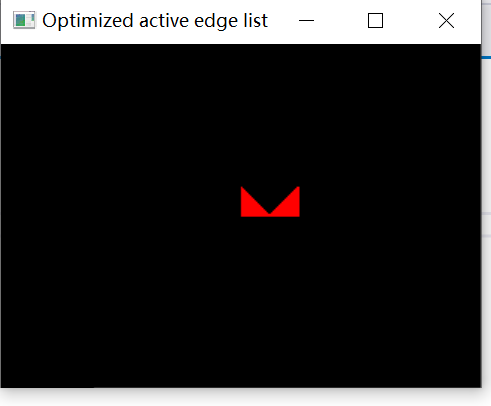### 二、算法分析+代码思想简述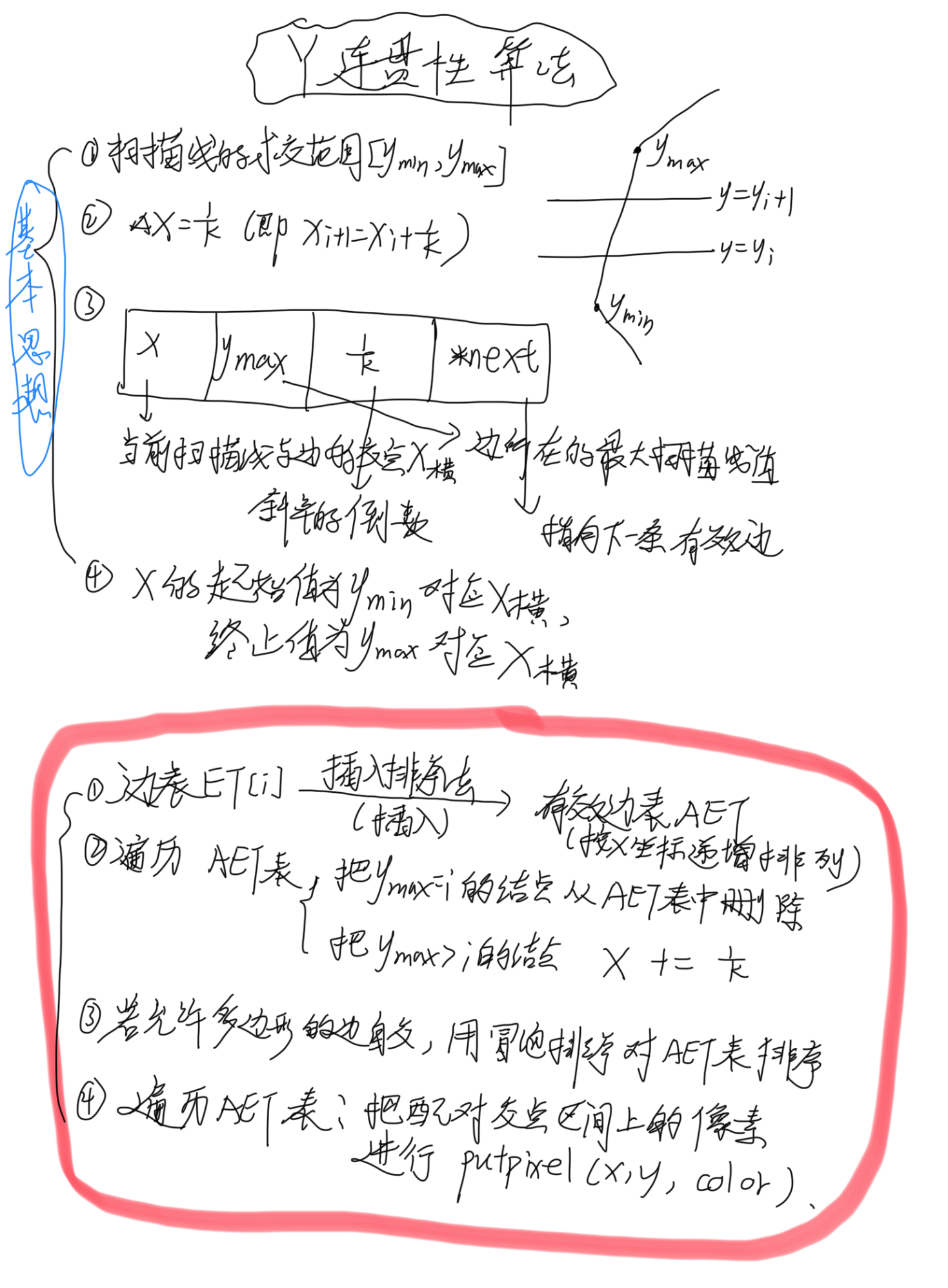### 三、例子图解

（注：引自老师课上的PPT）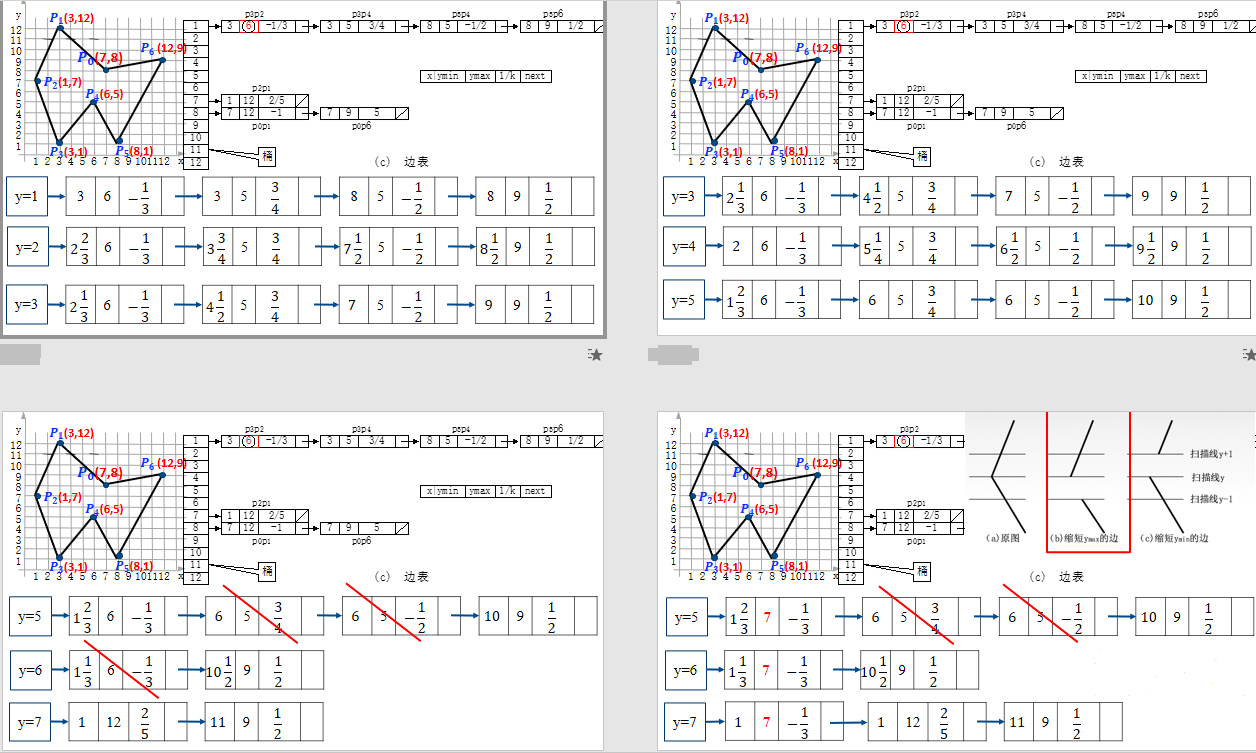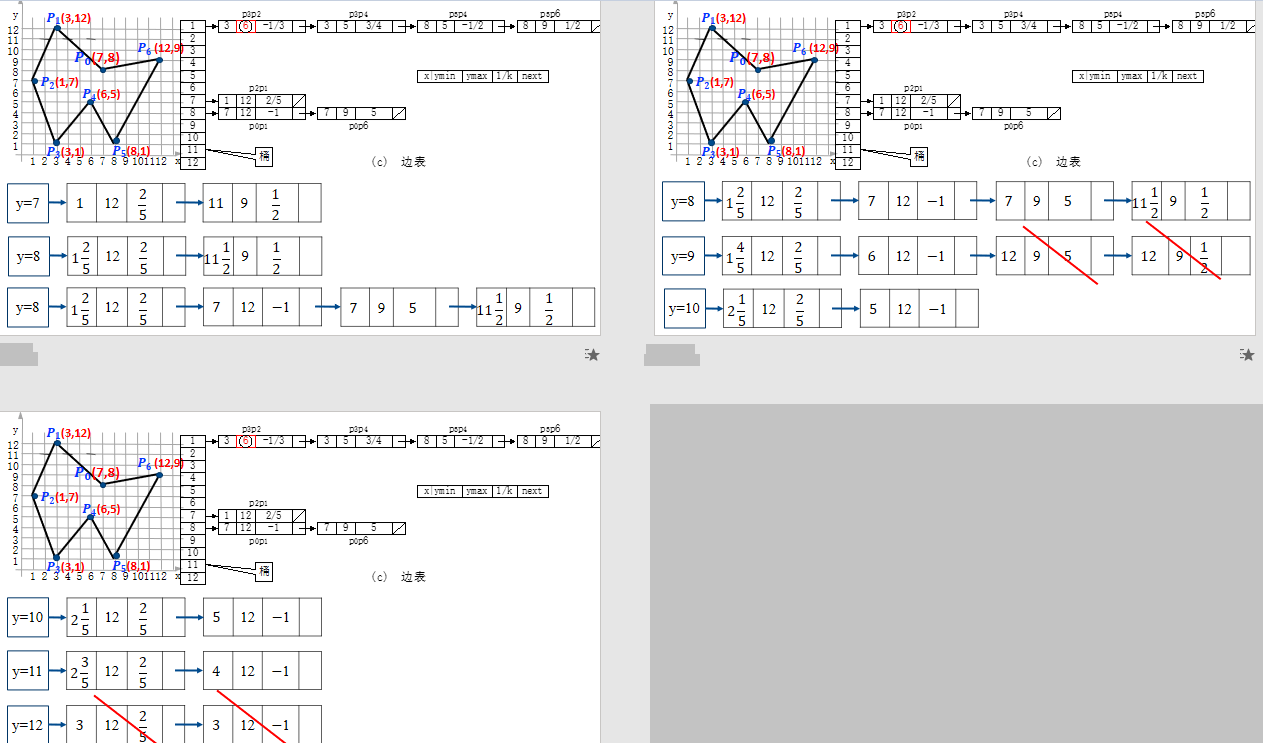展开全文• 使用MFC编写的多边形有效边表填充算法，是完整的算法 大家有需要的借鉴借鉴
• 针对现有基于UWB的井下定位算法存在算法复杂且求解值不是全局最优的问题，提出了一种基于UWB测距的三定位改进算法。该算法基于DW1000芯片和三定位算法，采用双边双向测距方法测距，以求解二元二次方程最优解为...
• 为提高区域填充效率，对三种常见的区域填充算法进行了介绍和分析，并对其中优势较为明显的活性边表区域填充算法进行了进一步改进改进算法针对原始算法的不足，充分利用多边形顶点信息，建立了活性动态发现机制，...
• 关于最小成本生成树这是一个非常受欢迎的问题，以简洁快速的方式解决它具有重大的现实和... 最后，由于减少了时间复杂度，并且处理更加方便，因此可以得出结论，改进的Kruskal算法在大多数情况下比Kruskal算法有效
• TL-SLM算法有效减少了带信息的传输，但PA P R的降低幅度有限。因此，进一步提出基于旋转向量的改进TL-SLM算法，即TR-SLM算法，该算法通过旋转向量的引入，产生更多的时域备选信号，达到进一步降低PA P R的目的。...正交频分复用 混沌序列 旋转向量
• 针对粒子群算法整体上容易陷入局部最优的缺陷，将鱼群算法中的视距、拥挤度引入标准粒子群算法，提出一种改进的粒子群算法有效提高了粒子群算法的全局收敛性。通过基准函数Sphere、Griewank、Ackley和Shekel’s ...粒子群优化
• 针对鲸鱼优化算法(whale optimization algorithm, WOA)容易陷入局部最优和收敛精度低的问题进行了研究，提出一种改进的鲸鱼优化算法(IWOA)。该算法通过准反向学习方法来初始化种群，提高种群的多样性；然后将线性...

# 一、理论基础

请参考这里

## 2、改进的鲸鱼优化算法

基本的鲸鱼优化算法仍然存在着求解精度低、收敛速度慢和易陷入局部最优的缺点。为了克服这些缺点，本文将从种群初始化、位置更新策略以及预防陷入局部最优这三个方面对WOA进行改进。

请参考这里

### （2）非线性收敛因子

由于收敛因子 a a 进行线性变化并不能很好地调节全局搜索能力和局部开发能力，因此本文提出来一种非线性收敛因子为 a = 2 − 2 sin ⁡ ( μ t m a x _ i t e r π + φ ) (1) a=2-2\sin(\mu\frac{t}{\rm{max\_iter}}\pi+\varphi)\tag{1} 其中， m a x _ i t e r \rm{max\_iter} 为最大迭代次数； t t 为当前迭代次数； μ \mu φ \varphi 是其表达式相关参数，选取 μ = 1 2 , φ = 0 \mu=\frac12,\varphi=0

### （3）自适应权重策略与随机差分法变异策略

鲸鱼优化算法在后期局部开发时易陷入局部最优，出现早熟收敛的现象，为了使算法能够保持种群的多样性并且能够及时跳出局部最优，提出来一种自适应权重策略和随机差分变异策略。
自适应权重策略数学表达式如下： ω = 1 − e t m a x _ i t e r − 1 e − 1 ,    X ( t + 1 ) = ω ⋅ X p ( t ) − A ⋅ D (2) \omega=1-\frac{e^{\frac{t}{\rm{max\_iter}}}-1}{e-1},\,\,\boldsymbol X(t+1)=\omega\cdot\boldsymbol X_p(t)-\boldsymbol A\cdot \boldsymbol D\tag{2} X ( t + 1 ) = ω ⋅ X p ( t ) + D ⋅ e b l ⋅ cos ⁡ ( 2 π l ) (3) \boldsymbol X(t+1)=\omega\cdot\boldsymbol X_p(t)+\boldsymbol D\cdot e^{bl}\cdot\cos(2\pi l)\tag{3} 随机差分变异策略如下： X ( t + 1 ) = r 1 × ( X p ( t ) − X ( t ) ) + r 2 × ( X ′ ( t ) − X ( t ) ) (4) \boldsymbol X(t+1)=r_1\times(\boldsymbol X_p(t)-\boldsymbol X(t))+r_2\times(\boldsymbol X'(t)-\boldsymbol X(t))\tag{4} 其中， r 1 r_1 r 2 r_2 均为 [ 0 , 1 ] [0,1] 的随机数； X ′ ( t ) \boldsymbol X'(t) 为种群中随机选取的个体。
每个个体都要经过包围捕食、螺旋更新、搜索猎物阶段，当个体进行包围捕食或螺旋更新时采用自适应权重策略去更新位置，之后个体需要通过随机差分变异策略对其再次更新，取其变化前后的最优位置，加快了种群的收敛，有效地防止了种群陷入局部最优。种群通过这两种策略协同工作，使得算法具有更好的寻优效果。

# 二、仿真实验及分析

将IWOA与WOA、PSO和GSA算法进行对比，实验设置迭代次数均为500次，所有算法种群规模均为30，以文献中表1列出的9个测试函数为例，每个算法独立运行30次，结果显示如下：函数：F1
WOA：最差值: 6.0434e-74, 最优值: 1.244e-83, 平均值: 4.8524e-75, 标准差: 1.5191e-74, 秩和检验: 1.2118e-12
PSO：最差值: 1844.6136, 最优值: 319.9645, 平均值: 897.9079, 标准差: 362.3394, 秩和检验: 1.2118e-12
GSA：最差值: 0.0048082, 最优值: 1.0156e-16, 平均值: 0.00016027, 标准差: 0.00087785, 秩和检验: 1.2118e-12
IWOA：最差值: 0, 最优值: 0, 平均值: 0, 标准差: 0, 秩和检验: NaN
函数：F2
WOA：最差值: 6.5023e-49, 最优值: 4.0384e-59, 平均值: 3.1087e-50, 标准差: 1.2591e-49, 秩和检验: 3.0199e-11
PSO：最差值: 49.9354, 最优值: 17.0827, 平均值: 25.3533, 标准差: 6.1061, 秩和检验: 3.0199e-11
GSA：最差值: 6.4411, 最优值: 6.1151e-08, 平均值: 0.7822, 标准差: 1.3817, 秩和检验: 3.0199e-11
IWOA：最差值: 5.0507e-300, 最优值: 5.6662e-313, 平均值: 1.7001e-301, 标准差: 0, 秩和检验: 1
函数：F3
WOA：最差值: 86803.9677, 最优值: 10152.6554, 平均值: 41769.2155, 标准差: 15822.3354, 秩和检验: 1.2118e-12
PSO：最差值: 12063.1708, 最优值: 2237.6953, 平均值: 6498.1617, 标准差: 2843.8074, 秩和检验: 1.2118e-12
GSA：最差值: 1797.8533, 最优值: 258.558, 平均值: 947.1683, 标准差: 360.8248, 秩和检验: 1.2118e-12
IWOA：最差值: 0, 最优值: 0, 平均值: 0, 标准差: 0, 秩和检验: NaN
函数：F4
WOA：最差值: 89.3781, 最优值: 0.10516, 平均值: 56.2233, 标准差: 27.7756, 秩和检验: 3.0199e-11
PSO：最差值: 14.9822, 最优值: 6.586, 平均值: 10.8541, 标准差: 1.9351, 秩和检验: 3.0199e-11
GSA：最差值: 12.3007, 最优值: 4.3621, 平均值: 7.4295, 标准差: 2.0443, 秩和检验: 3.0199e-11
IWOA：最差值: 2.8436e-299, 最优值: 9.7449e-310, 平均值: 1.2816e-300, 标准差: 0, 秩和检验: 1
函数：F5
WOA：最差值: 0.017149, 最优值: 2.1308e-05, 平均值: 0.0034382, 标准差: 0.0041751, 秩和检验: 3.4742e-10
PSO：最差值: 3.997, 最优值: 0.55255, 平均值: 1.6619, 标准差: 0.88162, 秩和检验: 3.0199e-11
GSA：最差值: 3.3787, 最优值: 0.044496, 平均值: 0.28219, 标准差: 0.59797, 秩和检验: 3.0199e-11
IWOA：最差值: 0.00019378, 最优值: 2.8772e-06, 平均值: 4.8017e-05, 标准差: 4.4012e-05, 秩和检验: 1
函数：F6
WOA：最差值: -7519.4512, 最优值: -12568.8221, 平均值: -10424.1727, 标准差: 1867.7742, 秩和检验: 7.0881e-08
PSO：最差值: -2168.2069, 最优值: -3829.459, 平均值: -2904.2623, 标准差: 396.5414, 秩和检验: 3.0199e-11
GSA：最差值: -2184.4459, 最优值: -4408.3773, 平均值: -2727.2632, 标准差: 494.7846, 秩和检验: 3.0199e-11
IWOA：最差值: -11287.5412, 最优值: -12569.486, 平均值: -12465.7503, 标准差: 279.0951, 秩和检验: 1
函数：F7
WOA：最差值: 5.6843e-14, 最优值: 0, 平均值: 1.8948e-15, 标准差: 1.0378e-14, 秩和检验: 0.33371
PSO：最差值: 180.5696, 最优值: 113.3323, 平均值: 144.9122, 标准差: 18.0355, 秩和检验: 1.2118e-12
GSA：最差值: 55.7174, 最优值: 21.8891, 平均值: 33.2979, 标准差: 8.4858, 秩和检验: 1.2098e-12
IWOA：最差值: 0, 最优值: 0, 平均值: 0, 标准差: 0, 秩和检验: NaN
函数：F8
WOA：最差值: 7.9936e-15, 最优值: 8.8818e-16, 平均值: 3.7303e-15, 标准差: 2.7041e-15, 秩和检验: 7.4605e-07
PSO：最差值: 10.2481, 最优值: 6.2133, 平均值: 8.0176, 标准差: 1.0408, 秩和检验: 1.2118e-12
GSA：最差值: 0.9313, 最优值: 8.0807e-09, 平均值: 0.031043, 标准差: 0.17003, 秩和检验: 1.2118e-12
IWOA：最差值: 8.8818e-16, 最优值: 8.8818e-16, 平均值: 8.8818e-16, 标准差: 0, 秩和检验: NaN
函数：F9
WOA：最差值: 0.15452, 最优值: 0, 平均值: 0.0051507, 标准差: 0.028212, 秩和检验: 0.33371
PSO：最差值: 24.8604, 最优值: 7.3018, 平均值: 13.0565, 标准差: 4.9294, 秩和检验: 1.2118e-12
GSA：最差值: 38.4571, 最优值: 16.8362, 平均值: 26.7235, 标准差: 6.1123, 秩和检验: 1.2118e-12
IWOA：最差值: 0, 最优值: 0, 平均值: 0, 标准差: 0, 秩和检验: NaN


实验结果表明：相比其他优化算法和基本鲸鱼优化算法，改进的鲸鱼优化算法其收敛精度最高，算法稳定性最好。

# 三、参考文献

 武泽权, 牟永敏. 一种改进的鲸鱼优化算法[J]. 计算机应用研究, 2020, 37(12): 3618-3621.

展开全文matlab
• ## 粒子群算法及其改进算法

万次阅读 多人点赞 2019-07-01 19:27:08
标准粒子群算法及其改进算法 首先在这里介绍一下，这个里主要介绍粒子群算法以及一个改进的二阶振荡粒子群算法。 原理 粒子群优化（PSO）算法是Kennedy和Eberhart受 鸟群群体运动的启发于1995年提出的一种新的群...

# 标准粒子群算法及其改进算法

首先在这里介绍一下，这个里主要介绍粒子群算法以及一个改进的二阶振荡粒子群算法。

## 原理

粒子群优化（PSO）算法是Kennedy和Eberhart受 鸟群群体运动的启发于1995年提出的一种新的群智能优化算法。大概的意思就是一片森林里有一群鸟在找一块食物，它们不知道食物具体在哪，但是可以通过感官（例如嗅觉）去察觉到自己当前位置距离食物的远近。鸟可以记住自己走过的位置并且知道自己做过的最优位置。这一群鸟的运动都是随机的，这类似于一种穷举法。

## 标准粒子群算法

粒子群算法一般用来找一个函数的最优值。这个函数一般就是适应度函数。
函数中未知量的个数就是这个查找的空间维度。

### 接下来种群中每个粒子按照公式更新速度和位置：

Vi( t +1 ) =w * Vi( t ) + c1 * r1 * (pi - xi( t ) ) + c2 * r2 * ( pg - xi( t ) ) （1）
xi( t + 1 ) = xi( t ) + vi( t + 1 ) （2）

PS：这里的r1、r2是每一步迭代都需要更新的随机数
c1、c2和w =则是一开始给定的一些参数，至于参数的给定取决于你自己每次测试这个程序所得到的经验–即哪些参数你跑出的结果比较好就选择哪些参数。
在这里再插一句，在我的实践中认为没有必要去限制迭代过程中速度和位置是否超过最大值，如果在给定区间内存在最优值那么最后还是会迭代回来。只需要在给定的区间内初始化就OK了。在我最开始的测试过程中限制速度和位置是使程序变慢了的，但是我一开始的思路出了问题，到很后面才改正过来也么有再去测试这个，所以就不加评论了。

## 算法流程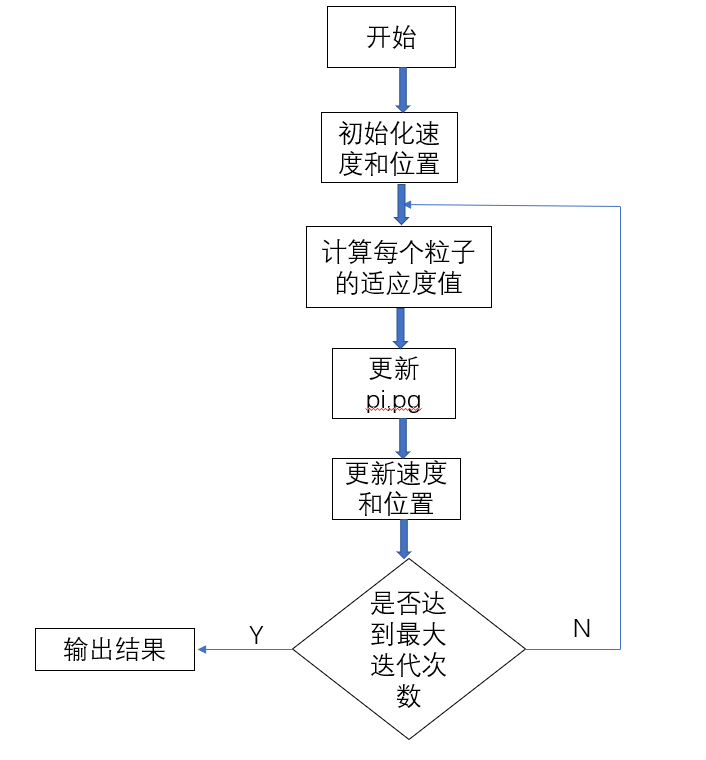### 标准PSO算法代码

function [xm,fv]=Pso(N,c1,c2,w,M,D)
%c1:自我学习因子
%c2:社会学习因子

%w:惯性权重
%N:种群数量
%M:最大迭代次数
%D:维数

%初始化种群、速度和位置
tic
for i=1:N
for j=1:D
x(i,j)=unifrnd(-10,10);
v(i,j)=unifrnd(-10,10);
end
end

%根据适应度计算适应度值,初始化pi和pg
for i=1:N
y(i)=test(x(i,:));
pi(i,:)=x(i,:); %y(i,:)为最优位置
end
k = find( y == min(y) ) ;
pg=x(k(1), : ) ;
%更新位置与速度
for t=1:M
for i=1:N
v(i,:)=wv(i,:)+c1rand*(pi(i,:)-x(i,:))+c2rand(pg-x(i,:));
x(i,:)=x(i,:)+v(i,:);
if test(x(i,:))<y(i)
y(i)=test(x(i,:));
pi(i,:)=x(i,:);
end
if y(i)<test(pg)
pg=pi(i,:);
end
end
pbest(t)=test(pg);
end

%输出结果
disp(‘最优位置’);
xm=pg
disp(‘最优值’);
fv=test(pg)
plot(pbest)
toc

### test函数–就是适应度函数

function y = test( V )
y=0;
b=length(V);
for i=1:b
y=y+i*V(i)^2;
end

## 标准粒子群算法的局限性

为进一步说明标准粒子群算法的局限性，做如下推理：
设 φ1=c1*r1 ，φ2=c2r2 ，w=1，由式（1）、（2）可转化 为式（3）、（4）：
Vi( t+1) =Vi( t) +φ1(pi -xi(t))+φ2(pg -xi(t)) （3）
xi(t+1)=xi(t)+vi(t+1) （4）
已知速度公式Vi+1=Vi +a×Δt ，可得： a=φ1(pi -xi(t))+φ2(pg -xi(t))=xi(t)″ （5）
化简可得： xi(t)″+(φ1+φ2)xi(t)-(φ1pi +φ2pg)=0 （6）
式（6）是二阶微分方程，通过二阶微分方程求解公式，求得搜索位置在 t 代的 x(t)的位置路径公式： xi(t)=C1 cos( φ1+φ2t)+C2 sin( φ1+φ2t) （7）
由式（7）可知，xi(t) 在一个固定区间内波动，即在 该区间中寻找每个粒子第 t 次迭代后的位置，位置函数 xi(t) 没有振荡收敛，算法容易陷入局部最优。
例如，令 C1=1,C2=1, φ1+φ2 =1 ，可得位置函数 xi(t)=cos(t)+ sin(t)的图像，如图1所示。在该图像中， 对于t∈[ -∞，+∞] ， xi(t) 始终在固定上下限 [- 2, 2] 内范围波动，没有振 荡收敛，容易陷入局部最优。因此，在算法中加入振荡 收敛，是跳出局部最优解，提高粒子群算法搜索性能和精度较有效的方法。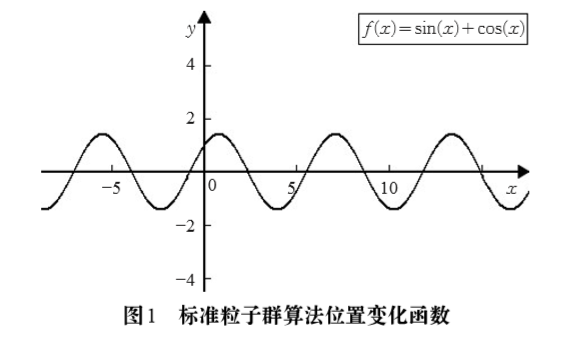### 改进标准粒子群算法的思想

胡建秀，曾建潮通过在标准二阶粒子群算法速度迭 代方程中引入二阶振荡环节的方法改进算法，来增加粒 子的多样性，提高算法的全局搜索能力，是改进位置函 数搜索区域较好的改进方法。使用二阶振荡环节后，算法前期具有较强的全局搜索能力，振荡收敛；而在后期强局部搜索，渐近收敛。 该粒子群算法的进化方程如下：
Vi( t+1) =w×Vi( t ) + φ1(pi -(1+ξ1)xi(t)+ξ1xi(t-1))+ φ2(pg -(1+ξ2)xi(t)+ξ2xi(t-1)) （8） xi(t+1)=xi(t)+vi(t+1) （9） 算法振荡收敛：
ξ1<2 φ1 -1 φ1 ，
ξ2<2 φ2 -1 φ2 （10）
算法渐进收敛：
ξ1 ≥2 φ1 -1 φ1 ，
ξ2 ≥2 φ2 -1 φ2 （11） 振荡收敛和渐进收敛示意图如图2和图3所示。[ 1 ]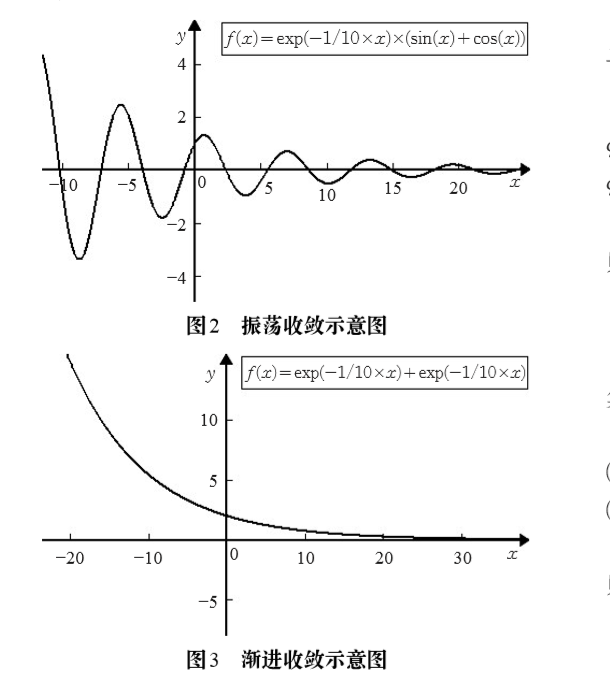### 改进后二阶振荡粒子群算法的迭代公式

Vi( t+1 ) =w×Vi( t ) + φ1(pi -(1+ξ1)xi(t)+ξ2xi(t-1))+ φ2(pg -(1+ξ3)xi(t)+ξ4xi(t-1)) （12） xi(t+1)=xi(t)+vi(t+1)

这个公式的实在上面的二阶振荡粒子群算法的基础上进行的改进，这里ξ虽然是随机数但是取值是有限制的：
设最大迭代次数为Gmax ,
0<ξ2<1+ξ1 2 ,0<ξ4 <1+ξ3 2 ,0<ξ1<1,0<ξ3<1 当 t < Gmax / 2时，
ξ1<ξ2-1,ξ3<ξ4 -1,0<ξ2<1,0<ξ4 <1 当 t > Gmax / 2 时
这么做的意义在于可以时算法在迭代的前半段振荡收敛；
在迭代的后半段使其渐进收敛。
这里的证明和上面的二阶振荡粒子群算法的类似，我这就不展开了，感兴趣的可以自己去找我参考的文献。
PS：关于改进算法的流程图和标准算法的类似，无非就是加了一个迭代次数前一半和后一半参数的改变，这里就不加上去了。

### 改进二阶振荡粒子群算法代码

function [ z , best ] = PSO_1( w , Gmax ,lizishu , weidu ,a , b )
%这个测试函数的最小值是0，取值范围是[-10 , 10]
% z是最优点的适应值，best是记录最优位置的坐标

%lizishu输入需要的粒子数
%weidu函数的自变量个数
%a下界
%b上界
q=zeros(1,Gmax);
tic
p = inf * ones( 1 , lizishu ) ;%初始的最优解默认为inf
% pg = 0 ;
A = unifrnd( a , b , lizishu , weidu ) ;%A矩阵是位置矩阵，用于储存每一步计算出的位置
B = unifrnd( a , b , lizishu , weidu ) ;%B是速度矩阵，用于储存每一布计算出的V
C = A ;%用于记录每个粒子的最优位置
D = unifrnd( a , b , lizishu , weidu ) ;%位置矩阵，用于记录上一次迭代的位置
c = 2 ; %c通常取1.5 ， 这是一个经验值
y = [] ;%给y申请空间
for i = 1 : lizishu
y = [ y , test( A( i ,: ) ) ] ;
end

for i = 1 : lizishu
if p( i ) > y( i )
C( i , : ) = A( i , : ) ;
end
p( i ) = min( [ p( i ) , y( i ) ] ) ;%更新局部最优解
end

%初始化全局最优解
k = find( p == min( p ) ) ;
pg = C( k( 1 ) , : ) ;
q( i ) = test( pg ) ;

for i = 1 : Gmax
i
r1 = unifrnd( 0 , 1 ) ;
r2 = unifrnd( 0 , 1 ) ;
u1 = r1 * c ;
u2 = r2 * c ;
if i < Gmax / 2
g1 = unifrnd( 0 , 1 ) ; % 这里g1是[ 0 , 1 ]上均匀分布的随机数
g2 = ( 1 + g1 ) / 2 ;
g3 = unifrnd( 0 , 1 ) ;
g4 = ( 1 + g3 ) / 2 ;
for j = 1 : lizishu
B( j , : ) = w * B( j , : ) + u1 * ( C( j , : ) - ( 1 + g1 ) * A( j , : ) + g2 * D( j , : ) ) + u2 * ( pg - ( 1 + g3 ) * A( j , : ) + g4 * D( j , : ) ) ;
D = A ;
A( j , : ) = A( j , : ) + B( j , : ) ;
end
else
g2 = unifrnd( 0 , 1 ) ; % 这里g2是[ 0 , 1 ]上均匀分布的随机数
g1 = ( g2 - 1 ) / 4 ;
g4 = unifrnd( 0 , 1 ) ;
g3 = ( g4 - 1 ) / 4 ;
for j = 1 : lizishu
B( j , : ) = w * B( j , : ) + u1 * ( C( j , : ) - ( 1 + g1 ) * A( j , : ) + g2 * D( j , : ) ) + u2 * ( pg - ( 1 + g3 ) * A( j , : ) + g4 * D( j , : ) ) ;
D = A ;
A( j , : ) = A( j , : ) + B( j , : ) ;
end
end
y = [] ;%下一次迭代前清空y
for j = 1 : lizishu
y = [ y , test( A( j ,: ) ) ] ;
end

   for j = 1 : lizishu
if p( j ) > y( j )
C( j , : ) = A( j , : ) ;
end
p( j ) = min( [ p( j ) , y( j ) ] ) ;
end

k = find( p == min( p ) ) ;%找到最优解的位置
pg = C( k( 1 ) , : ) ; %保存最优解坐标
q(i) = test( pg ) ;
`

end
z = q( Gmax ) ;
best = pg ;
toc
plot(q)

### test函数

function y = test( V )
y=0;
b=length(V);
for i=1:b
y=y+i*V(i)^2;
end

### 两个算法的比较

PS:上面我只给了一个test函数,要测试其他的函数直接更改test函数即可。
下面是两个维度跑出来的结果
1、标准PSO算法：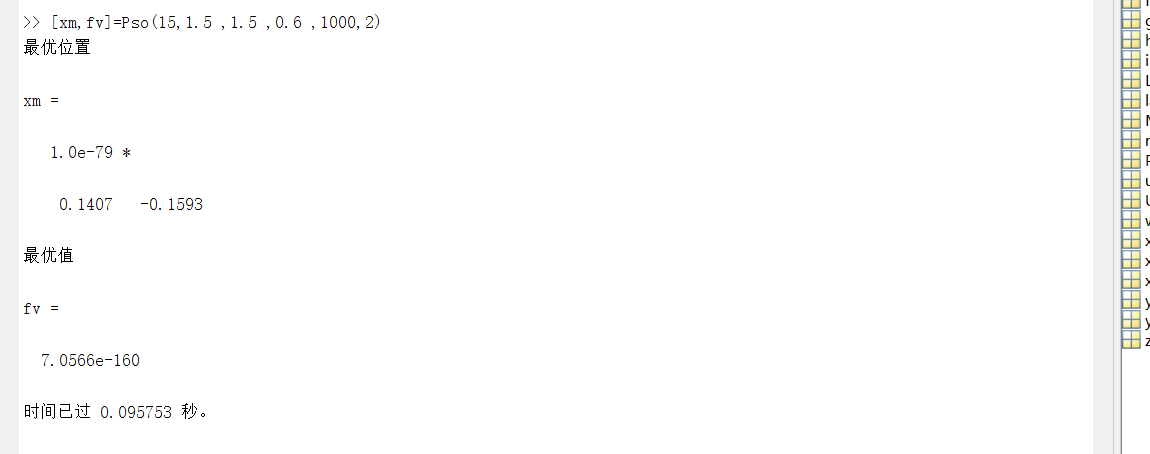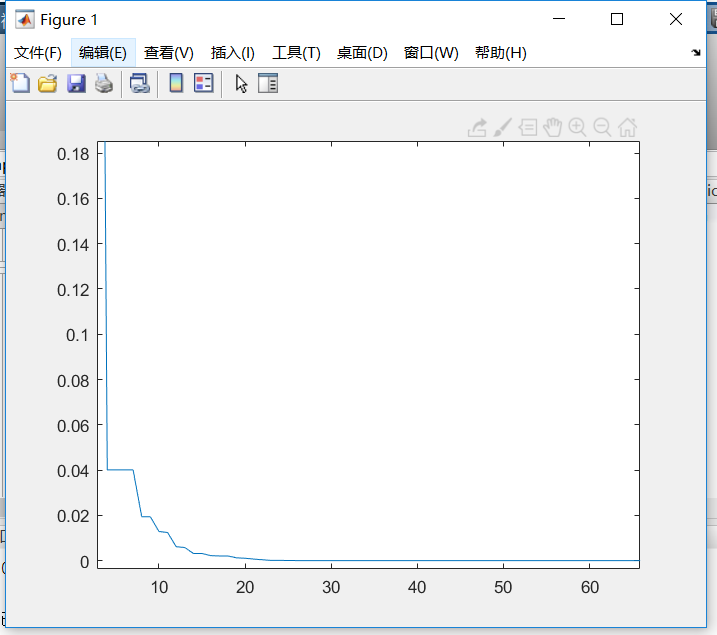2、改进的二阶振荡PSO算法: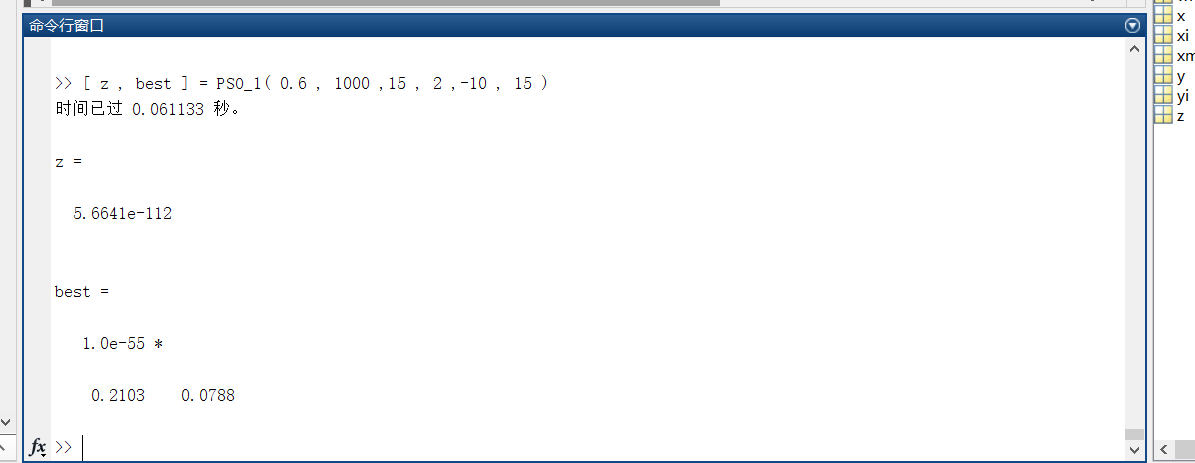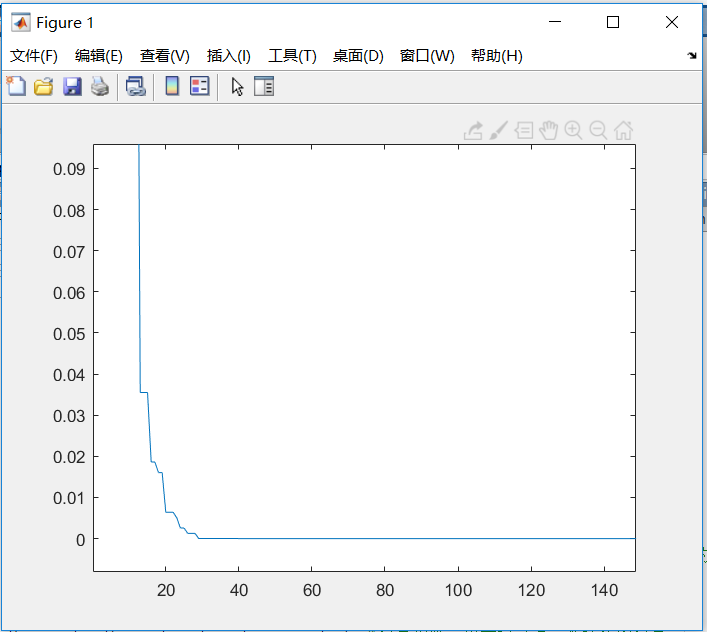在低维度上这两个算法没有太大差别，改进的算法速度上要稍微快一点。

下面把维度提升到100维：
PS：为了便于观看我改了一下程序，最后都只输出一个最优值。
1、这是标准PSO算法跑出的结果：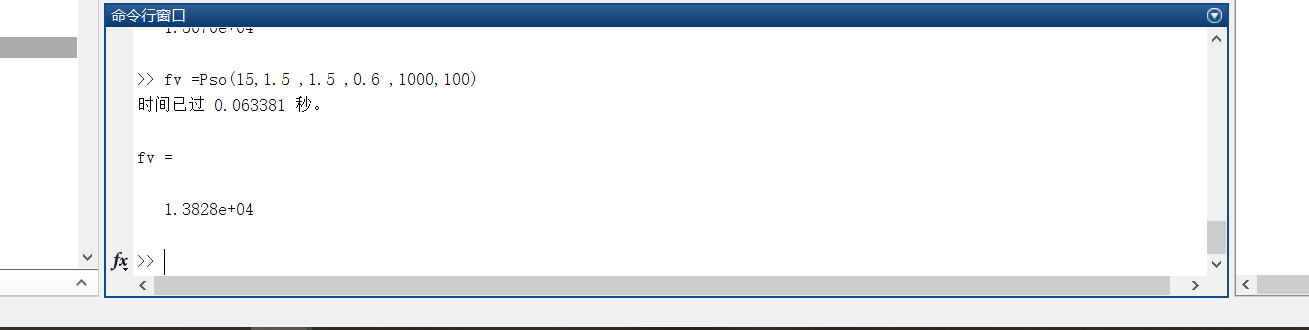很明显这并没有达到最优值，只是一个局部最优。

2、改进的PSO算法：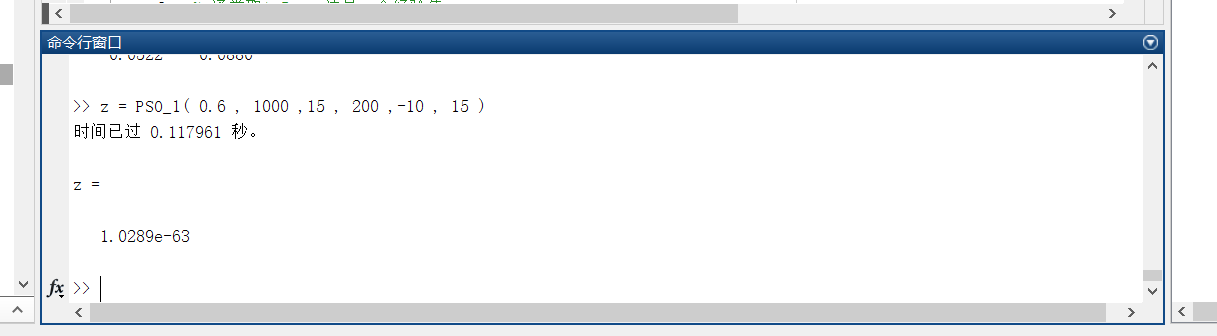可以看到改进的算法的结果在100维下依旧不错，而且很快。

下面我们尝试1000维：
改进的PSO算法结果如下：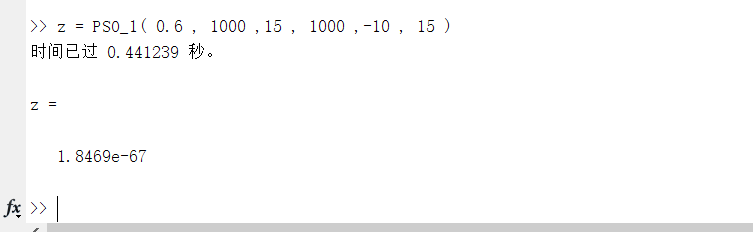我也试过一些最小值是无穷的函数（X^3），以及一些振荡函数(sinx+cosx),都可以跑出结果，这里就不一个个的给出结果了。

PS：因为第一个算法不是我写的原因，是我同学写的我拿来用的，所以两个代码在风格上差别有点大。
这个博客的证明部分基本上我都是从下面的文献里直接拿过来的。
最后如果找出我的错误请告诉我，我会及时改正的。

### 参考文献

[ 1 ] 蒋丽,叶润周，梁昌勇等，改进的二阶振荡粒子群算法[J]，计算机工程与应用,2009，55（9）：130-139

展开全文• 文章目录一、理论基础1、鲸鱼优化算法2、混合策略改进的鲸鱼优化算法（1）非线性收敛因子（2）自适应权重系数（3）limit阈值二、仿真结果三、参考文献四、Matlab仿真程序 一、理论基础 1、鲸鱼优化算法 请参考这里。...matlab 函数寻优
• 1、活性边表（AET）：把与当前扫描线相交得称为活性，并把它们按与扫描线交点x坐标递增得顺序存放在一个链表中。 2、结点内容（一个结点在数据结构里可用结构来表示） x：当前扫描线与得交点坐标 Δx：从...
• 针对以高效求解有数限制的最短路问题，对经典Bellman-Ford算法进行了改进．借鉴划分算法的思想，通过减少...相对于经典Bellman-Ford算法改进后的算法不仅可有效地节省存储空间，而且实验表明能显著地提高计算效率．
• 针对NP-hard性质的作业车间调度问题, 设计了一种改进的离散粒子群优化算法。引入遗传算法交叉算子和变异算子来...同时, 将该算法结果与文献中其他相关算法结果进行比较, 验证了该改进算法有效性。该算法能够有效
• 文章目录一、理论基础1、基本灰狼优化算法2、改进灰狼优化算法（1）佳点集种群初始化（2）非线性控制参数策略（3）基于个体记忆功能的位置更新公式二、仿真实验与分析三、参考文献四、Matlab仿真程序 一、理论基础 1...matlab
• ## 改进算法的方法

千次阅读 2019-05-12 18:34:02
该方法可以改进高方差问题，从学习曲线可以看出，随着样本量的增加，交叉验证误差和训练集误差越来越接近 2.减少特征 该方法可以改进高方差问题，高方差是过拟合的情况，花时间去选取更少，更合适的的特征 3.增加...
• 针对黑猩猩优化算法存在易陷入局部最优、收敛速度慢、寻优精度低等缺陷，提出了混合改进策略的黑猩猩优化算法(SLWChOA)。首先，利用Sobol序列初始化种群，增加种群的随机性和多样性，为算法全局寻优奠定基础；其次，...matlab
• 盖师贝格-撒克斯通（GS）算法及其改进算法 本文摘自李景镇《光学手册》 盖师贝格(R.W.Gerchberg)和撒克斯通(W.O.Saxton)首先提出了一种振幅相位恢复算法，即GS算 法，利用输入输出间的正反傅里叶变换与输入输出面上...衍射光学
• ## 改进算法1

千次阅读 2019-03-08 10:56:51
当训练好一个模型之后预测新的数据，当发现预测情况不是很好的时候，怎么改进？ 1.得到更多的训练数据。但有的时候获取更多的数据并不是很有帮助 ...评估该算法的性能（机器学习诊断法），从而能知道影响性能的...
• 通常改进算法性能有以下几种方法： 1、增加数据 但是有更多的数据不一定能获得更好的效果。 2、选用更少的特征 来防止过拟合。 3、选用更多的特征 也许特征不足，会导致欠拟合。 4、增加额外的多项式特征 例如x12，...机器学习
• ## 蚁群算法简析、缺陷、改进

万次阅读 多人点赞 2020-07-18 18:20:56
蚁群算法是一种用来寻找优化路径的概率型算法，模拟蚂蚁在寻找食物过程中发现路径的行为。人工智能 python 大数据
• 今天我们赏析一篇高引用的中文论文——《应用Otsu改进Canny算子的图像边缘检测方法》。 是的，这是一篇2014年发表于<计算机与数字工程>的论文，作者是顺德职业技术学院的张志强教授和中国科学院研究生院的宋...
• 群智能算法改进思路 本文仅从方法论的角度来进行探讨，不设计具体的改进方法。 1.先看单个更新公式 我们常见的群智能算法其核心无非是位置更新公式，大多更新方式都可以写作下面的公式： X(t+1)=X′(t)+ΔX X(t+1) ...
• A*算法：Dijkstra改进算法A算法Dijkstra改进算法 寻路模型 A算法 算法简介 算法步骤 格点模型 格点模型分析 改进思路方向 导航网络算法步骤及其优缺点 A算法启发函数的选取 伪代码 参考外链寻路模型最优路规划问题...函数...